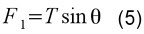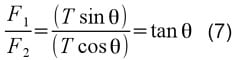In the late 18th century knowing the density of the Earth was a crucial, but missing, piece in a puzzle. Not only could that knowledge provide an insight in what was at the centre of the Earth, it could also provide the actual densities of every other body in the solar system – at this time only relative densities were known. This was important in working out whether they were rocky worlds like the Earth or predominately gassy planets.

Earlier, in the 17th century, Sir Isaac Newton had previously mulled over the possibility of using a mountain to test his theory of gravitational attraction. The idea went that if you put a pendulum near enough to a large mass – like a mountain – the deflection of the pendulum caused by the gravitational pull of the mountain might be enough to measure. Newton, however, never pursued the idea because he doubted whether the deviation would be sufficient.

The notion was picked up again by Astronomer Royal, Nevil Maskelyne in 1774 when he headed to Scotland to weigh the world. His assistants had located a kilometre high peak called Schiehallion (She-hal-e-on) which was deemed ideal due it being far from any other mountains and also because it was very symmetrical in shape. Maskelyne and his team set up camp on the mountain, staying there for 17 weeks, hampered a lot by rain, trying to make the appropriate measurements.

Here is a summary of what they were doing:

They hung a bob, of mass mb, on the end of string to make a pendulum a distance, d, away from the centre of mass of the mountain. The tension in the string was T. The bob experienced two other forces: a gravitational attractive force towards the mountain, F1, and a gravitational attractive force towards the Earth, F2. The force F1 pulled the bob towards the mountain causing the string to deviate slightly from the vertical by some angle, ?.

This is summed up by the following force diagram:From this we can start putting some equations together.

Newton’s law of gravitation says that:where Mm is the mass of the mountain and G is Newton’s Universal Gravitational Constant.

We can also say that:where ME and rE are the mass and radius of the Earth respectively.

Let’s take the ratio of the forces:The goal of the Schiehallion experimenters was a value for the density of the Earth. To get things in terms of density, mass needs to be substituted for density multiplied by volume. This gives:where ?m and Vm are the density and volume of the mountain. Similarly, ?E and VE are the density and volume of the Earth.

Because the string is in static equilibrium, the tension in the string, T, balances the forces F1 and F2. Resolving the components of the string tension gives two new equations:Taking the ratio of the two forces again gives:Equating (4) and (7) shows that:Re-arranging gives:The radius and volume of the Earth were known and the distance to the mountain was measurable. The density of the mountain was estimated by the density of the rock it was made from. The volume of the mountain was slightly more tricky. Maskelyne’s assistant, mathematician Charles Hutton, divided the mountain up into horizontal slices at regular intervals and worked out the volume of each slice. This was relatively straight forward as the mountain was chosen for its symmetrical nature. He then simply added the volume of each slice together to give the volume of the whole mountain. In doing so, he had invented the idea of contour lines still used to this day.

The only variable left to measure was the angle of deviation of the pendulum string and this is what took the most time and care. Being an astronomer Maskelyne naturally hit upon the idea of using the stars. The true vertical needed to be known incredibly accurately as the deviation from it was so small. Maskelyne used stars crossing the zenith, the point directly overhead, to establish a precise vertical line and then ? could be found.

Eventually after many measurements, and taking other factors into account, a value that is 75% of today’s accepted value of 5.5 grams per centimetre cubed was obtained. Here was evidence that the world could not be hollow, it was just too dense. The result also gave an approximate value of the density of every known solar system body and Newton’s theory of gravity had been vindicated. The world had been weighed, through mathematics, on a soggy mountain in Scotland.